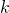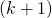# Projection algorithms for composite minimization

### Abstractcarpathian_2017_33_3_389_397_abstract

Issue no:

Parallel and cyclic projection algorithms are proposed for
minimizing the sum of a finite family of convex functions over the intersection
of a finite family of closed convex subsets of a Hilbert space. These algorithms
consist of two steps. Once the-th iterate is constructed, an inner circle of gradient descent process is executed through each local function, and then a parallel or cyclic projection process is applied to produce theiterate. These algorithms are proved to converge to an optimal solution of the composite minimization problem under investigation upon assuming boundedness of the gradients at the iterates of the local functions and the stepsizes being chosen appropriately.